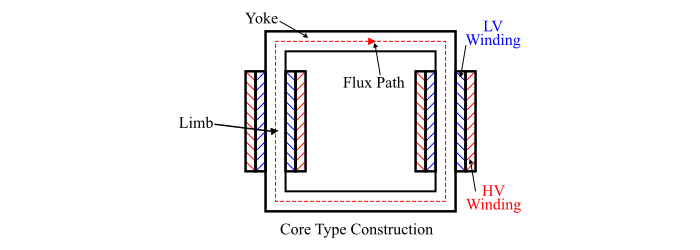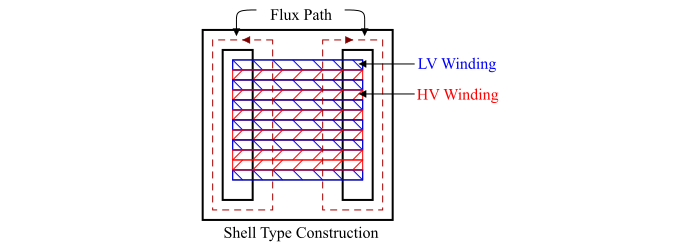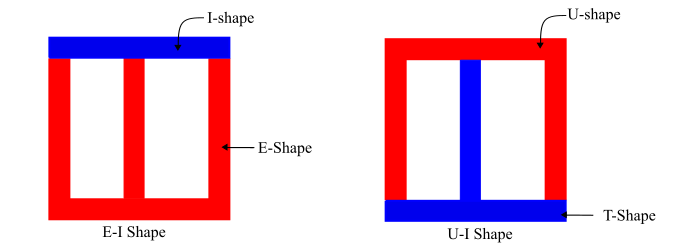# Single Phase Transformer ŌĆō Construction and Working Principle

## Construction of Single Phase Transformer

A single phase transformer consists of two windings viz. primary winding and secondary winding put on a magnetic core. The magnetic core is made from thin sheets (called laminations) of high graded silicon steel and provides a definite path to the magnetic flux. These laminations reduce the eddy-current losses while the silicon steel reduces the hysteresis losses.

The laminations are insulated from each other by enamel insulation coating. The thin laminations are stacked together to form the core of the transformer. The air-gap between the laminations should be minimum so that the excitation current being minimum.

For a single phase transformer, there are two types of transformer constructions viz. the core type and the shell type.

## Core Type Transformer Construction

In core type construction of the transformer, the magnetic circuit consists of two vertical lags (called limbs) and two horizontal sections called yokes. To minimise the effect of leakage flux, half of each winding is placed on each limb (see the figure).

The low-voltage winding is placed next to the core while the high-voltage winding over the low-voltage winding to reduce the insulation requirements. Therefore the two windings are arranged as concentric coils and known as cylindrical winding.The laminations of the core type transformer are of U-I shape as shown in the figure.## Shell Type Transformer Construction

In the shell type construction of transformer, the magnetic circuit consists of three limbs, both the primary and secondary windings are placed on the central limb and the two outer limbs complete the low reluctance flux path. The each winding is sub-divided into sections viz. the low voltage (LV) section and the high-voltage (HV) section, which are alternatively put one over the other in the form of sandwich (see the figure). Therefore, such windings are called sandwich winding or disc winding.The core of the shell type transformer is made up either U-T shape or E-I shape (see the figure).## Working Principle of Single Phase Transformer

The working of the transformer is based on the principle of mutual inductance between two coils wound on the same magnetic core.When an alternating voltage (V1) is applied to the primary winding, an alternating magnetic flux (Φm) sets up in the core and links with the secondary winding, i.e. the magnetic flux links both the windings of the transformer magnetically. This magnetic flux induces EMF E1 in the primary winding and E2 in the secondary winding according to Faraday’s law of electromagnetic induction.

According to Lenz’ law,

$$\mathrm{Primary\:EMF,E_{1}=-N_{1}\frac{d\phi_{m}}{dt}\:\:\:\:\:...(1)}$$\mathrm{Secondary\:EMF,E_{2}=-N_{2}\frac{d\phi_{m}}{dt}\:\:\:\:\:...(2)}$$Therefore,$$\mathrm{\frac{E_{2}}{E_{1}}=\frac{N_{2}}{N_{1}}\:\:\:...(3)}

From the above equations, it is clear that the induced EMFs in the primary and secondary windings depends upon the number of turn of the winding.

If ­Øæü1 > ­Øæü2, then ­ØÉĖ1 > ­ØÉĖ2 i.e. the primary EMF is greater than the secondary EMF, the transformer is called as step-down transformer.

If ­Øæü2 > ­Øæü1, then ­ØÉĖ2 > ­ØÉĖ1 i.e. the primary EMF is less than the secondary EMF, the transformer is called as step-up transformer.

If a load is connected across the terminals of the secondary winding, the secondary EMF causes a current I2 to flow through the load. In this way, a transformer transfers AC power from one circuit to another circuit with a change in voltage level without any electrical connection between both the circuits i.e. the power from input circuit to output circuit transfers magnetically. During this transfer of electrical power, the frequency does not change.